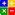# Ratio - Current RatioCURRENT RATIO:Online Current Ratio Calculator

This ratio is obtained by dividing the 'Total Current Assets' of a company by its 'Total Current Liabilities'. The ratio is regarded as a test of liquidity for a company. It expresses the 'working capital' relationship of current assets available to meet the company's current obligations. Therefore it is also known as the Working Capital.
When current assets equal current liabilities the ratio equals one. At this point the company has no working capital. A Current Ratio of over one means that the company has working capital. A ratio between 1.2 and 2 is deemed adequate. Anything over two can be argued as the company not being able to invest its excessive operational assets.

The formula:
Current Ratio = Total Current Assets/ Total Current Liabilities

DMC Firewall is a Joomla Security extension!